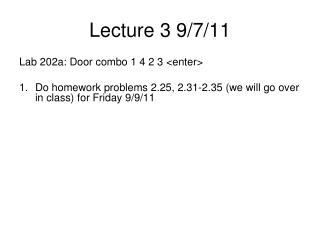DownloadDownload PresentationLecture 3 9/7/11

# Lecture 3 9/7/11

Télécharger la présentation## Lecture 3 9/7/11

- - - - - - - - - - - - - - - - - - - - - - - - - - - E N D - - - - - - - - - - - - - - - - - - - - - - - - - - -
##### Presentation Transcript

1. Lecture 3 9/7/11 Lab 202a: Door combo 1 4 2 3 <enter> • Do homework problems 2.25, 2.31-2.35 (we will go over in class) for Friday 9/9/11

2. Fixed Point Arithmetic • Floating point: overhead too high for many embedded apps – hundreds of operations to do FP ops • Fixed point: 2 part representation • Explicit variable integer I • Implicit (i.e. not stored, determined and remembered by programmer!) fixed constant  • Represented number F=I • Precision: number of distinguishable patterns, governed by number of bits in I • Resolution: smallest difference that can be represented,  by definition

3. Examples • Money! Integral pennies: =0.01 • Decimal-based implicit constant makes sense here – “Decimal fixed-point” though will be represented in binary of course • Voltmeter with resolution of 0.001V (i.e. integral mV) also makes sense: =0.001 • Decimal fixed point useful for managing displays to humans, binary fixed point where =2m is easier to computer with!

4. Examples • Voltmeter on 6811/12 • Built-in 8-bit ADC takes 0-5V input • 256 bit patterns to represent the entire range • Output N of the ADC is such that • Vin = 5*N/255 = 0.019607843*N • Smallest change we can detect is about 20mV • This one won’t discriminate between 3.005 and 3.008 V, or even 3.01 and 3.02 V • Design decisions • We now need to represent the voltage in a format convenient for display

5. Voltmeter design, cont. • Since a decimal display of voltage is the end game, we choose a decimal fixed point display with =0.01V • Why not =0.1V? • Why not =0.001V?

6. Voltmeter design, cont. • Since a decimal display of voltage is the end game, we choose a decimal fixed point display with =0.01V • Why not =0.1V? • Why not =0.001V? • Essential point: this resolution is a bit better than the raw ADC resolution (500 vs 256 patterns): we don’t want to lose resolution as an artifact of display format! Thermometers!!! • Complication: doesn’t fit in 8 bits! Need 916

7. Fixed point arithmetic • Main reason we use it: to add/subtract Fixed point numbers with same , just do integer add/subtract! • If numbers have different  values, we have to convert to a common basis first.

8. Fixed point arithmetic • we want z=x+y with x=I2n y=J2m z=K2p • algebraic manipulation gives K=I2n-p + J2m-p • alot like equalizing exponents in FP math

9. Fixed point arithmetic • multiplication, division more challenging • reasons similar to excess-bias problem in FP math • if you multiply two numbers representing dollar values stored as pennies (=0.01), straight multiplication gives the answer in terms of 1/10,000 dollars (1/100 of a penny) • need to multiply by 100 to get back to pennies representation

10. Freescale instruction set • Arithmetic, logical operaions • full complement of logical bitwise operators • AND, OR, NOT, XOR (EOR on Freescale) • details of instruction variants will follow in ch3 • 10 variants of AND instruction, for instance! • shift instructions • ASR, LSR; ASL, LSL; ROR, ROL • difference ASR,LSR? • difference ASL,LSL?

11. Arithmetic operations • There are integer instructions for + −×÷ • Challenge: programmer needs to check for anomalies such as overflow explicitly • Aid: CCR: Condition Code Register • unlike MIPS but like most other processors • 4 flags of interest to us now (there are more) • N negative: result of last computation was negative • Z zero: result of last computation was zero • V overflow: result of last computation was signed overflow (i.e. 2’s complement) • C carry: result of last computation was unsigned overflow • Each operation sets appropriate flag bits, programmer then checks them EVERY TIME!

12. Unsigned addition sequence • For example, knowing we just did an unsigned add (bcc = Branch if C flag Clear) ldaa A8 get first input adda B8 regA = A8 + B8 bcc OK1 if C=0 then no error so skip to end ldaa #255 overflow OK1 staa R8 R8= (smaller of A8+B8, 255) • Strategy here is to round an unsigned overflow back down to largest possible unsigned integer • Why not just abort? • What if V set?

13. Unsigned subtraction • similar idea: doing R8=A8-B8, set R8 to 0 on unsigned overflow (i.e. negative result)

14. Signed (2’s Comp) addition • The -128 – +127 problem complicates life a bit • several typos in your book on page 53 (at least in some printings) also complicate understanding • nb BMI is Branch if Minus, i.e. N=1. • BPL is Branch if Plus, i.e. N=0. • BVC is Branch if V flag Clear

15. ldaa A8 get first input adda B8 regA = A8 + B8 bvc ok3 if V=0 no error so skip to end err3 bmi over3 if V=1 and N=1, it was overflow ldaa #-128 if V=1 and N=0, it was underflow bra ok3 over3 ldaa #127 overflow ok3 staa R8 WAS MISSING IN BOOK